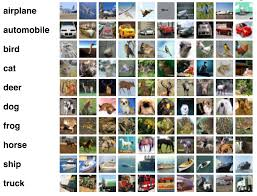# Image Classification Tutorial using K-Means in C#Source Code Link: Discover Groups – Similar Photos

In this tutorial we are going to build a simple image classifier. The only prerequisite is to have a good knowledge on K-Means clustering algorithm.

If you need a refresher you can check some of my other posts on K-Means:

And, if you would like to learn how to do image classification using ML.NET Model Builder, you can check out my latest post here:

How to use ML.NET Model Builder for Image Classification

## Image Classification

Grouping images into semantically meaningful categories using low-level visual features is a challenging and important problem in the area of Computer Vision.

We can define it as a process of assigning an input image with a label from a fixed set of categories.

So if we want to group these images using K-Means, first we need to choose the number of clusters. In order to do that we can use the “Elbow Method“. Or we can guess the correct K just from looking at the dataset.

Visually we can group these pictures in 4 categories.

• Blue underwater worlds
• White winter fairy tales
• Orange autumn nature

That is a quite easy task for a human. But how can we do the same type of grouping using K-Means?

## Feature Extraction

In machine learning and pattern recognition, a feature is defined as a measurable property or characteristic of the object you’re trying to analyze. That being said, we need to choose informative, discriminating and independent features that can be used for analysis.

One of the most prominent features in our dataset is the color. Meadows are green, deep water is blue, winter is best described by the white color and autumn is defined by the overly orange leaves.

As you can see feature extraction involves reducing the number of resources required to describe a large set of data. As a result, feature extraction is related to dimensionality reduction.

In this project we are not going to look at complex features. But just extract the color component of the image and classify it.

## RGB

In colored images, each pixel can be represented by a vector of three numbers (each ranging from 0 to 255) for the three primary color channels: red, green, and blue. These three red, green, and blue (RGBvalues are used together to decide the color of that pixel.

This means that our input vector will have three values. Therefore, we obtain the input vector with the following method:

``````double[] GetAverageRGB(Bitmap bmpImage)
{
double[] result = new double;
int numberOfPixlels = 0;

for (int i = 0; i < bmpImage.Width; i++)
{
for (int j = 0; j < bmpImage.Height; j++)
{
Color c = bmpImage.GetPixel(i, j);
result += c.R;
result += c.G;
result += c.B;
numberOfPixlels++;
}
}
result /= numberOfPixlels;
result /= numberOfPixlels;
result /= numberOfPixlels;
return result;
}``````

GetAverageRGB method is creating an input vector by calculating the mean over the RGB channels of the image.

As you can see from the dataset, all images have some dominant color. As a result, we are going to use the color component to find groups in the dataset.

The input for each image, will look something like this:

``Input Vector = [Mean R, Mean G, Mean B]``

After that, it’s K-Means job to train on that dataset and classify new images.

## K-Means

If you are unfamiliar with the K-Means clustering algorithm, you can check the following posts:

But, the main idea behind K-Means is to be able to cluster/group similar images by color. The expectation is that winter images will create a cluster, deep and underwater images will create it’s own and so on.

This notion is based on the feature extraction we did earlier. Since we are looking at the mean RGB for image, we can expect image grouping by color.

Now think about what other features are better and even more descriptive that will allow us even better clustering/grouping of our dataset.